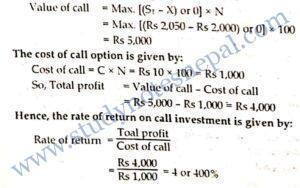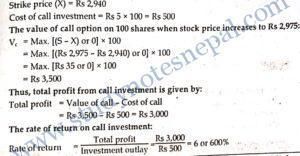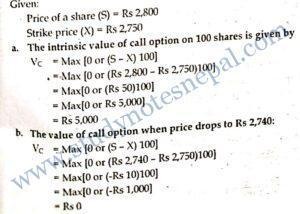###### 1. How a call option is different from put option?

A call option gives its holders the right, but not the obligation, to buy the underlying security at the exercise price, while a put option gives its holders the right, but not the obligation, to sell the underlying security at the exercise price. Call option becomes worthless when the value of underlying securities falls below the exercise price. In contrast, put option becomes worthless when the value of underlying securities increases above the exercise price.

###### 2. Differentiate between call option and put option.

A call option gives its holders the right, but not the obligation, to buy the underlying security at the exercise price, while a put option gives its holders the right, but not the obligation, to sell the underlying security at the exercise price. Call option becomes worthless when the value of underlying securities falls below the exercise price. In contrast, put option becomes worthless when the value of underlying securities increases above the exercise price.

###### 3. What do you mean by a call option?

A call option is the option that gives its holder the right to buy specified number of underlying securities at prespecified strike price within the stipulated time period.

###### 4. Define strike price of a put, option.

A put option gives holder the right to sell underlying assets at prespecified price within the stipulated time period. The prespecified price at which the holder can sell the underlying asset is called strike price of a put option.

NUMERICAL PROBLEMS

###### 5. XYZ stock is trading at Rs.300. Put options on this stock with strike price of Rs 400 are priced at Rs 105. What is the time premium on the put option? 

SOLUTION Given:

Selling price of stock (S) = Rs 300

Strike price of put (X) = Rs 400

Price of Put Option (P) = Rs 105

Now,

Intrinsic value of put (VP) = Max. [(X-S) or 0]

= Max. [(Rs 400 – Rs 300) or 0] = Rs 100

Time premium on the put = P – Vp = Rs 105 – Rs 100 = Rs 5

Hence, the time premium on the put option is Rs 5.

###### 6. Suppose the market price of an underlying stock is Rs 120. The strike price of call option on this stock is Rs 110. What is the value of a call option? What would be the value of option if it is a put?

SOLUTION

Given:

Selling price of stock (S) = Rs 120

Strike price on call (X) = Rs 110

The value of a call option is given by:

Vc = Max. [(S-X) or 0]

= Max. [(Rs 120 – Rs = Max. [Rs 10 or 0]

= Rs 10

The value of an option if it is put is given by:

Vp= Max. [(X-S) or 0]

= Max. [(Rs 110 – Rs 120) or 0]

= Max. [-Rs 10 or 0]

= Rs 0

###### 7. A put option has market price of Rs 15. The market price of underlying stock is Rs 200 and strike price of put is Rs 210. What is the premium on put option?

SOLUTION

Given:

Market price of put = Rs 15

Market price of stock (S) = Rs 200

Strike price of put (X) = Rs 210

Theoretical value of put option:

Vp = Max. [(X-S) or 0]

= Max. [(Rs 210 – Rs 200) or 0]

= Max. [Rs 10 or 0]

= Rs 10

Premium = Market price of put – Value of put = Rs 15 Rs 10 = Rs 5

###### 8. Supposé NEPSE index is soared upto 1500 points on certain date. What will be the value of index if the index is converted into cash at the scale of Rs 100 a point?

SOLUTION

Given:

NEPSE=1500 points

The cash value of index if the index is converted into cash at the scale of Rs 100 a point:

Cash value of index = 1,500 × Rs 100 = Rs 150,000

The main investment attraction of put and call options is the leverage benefit they offer. By making small investment in puts and calls, investors can earn higher rate of return on their investment if the price of underlying assets move in favorable direction. In other words, the leverage feature of puts and calls makes possible to earn high rate of return without investing large amount of money at the beginning. Large number of investors and speculators are attracted to put and call investments than the investment in underlying assets due to this attraction. However, puts and calls are also highly risky than the investment in underlying assets. The main risks associated with put and call investments are as follows:

1. Holders of calls and puts do not get dividend and interest and they did not have ownership right in the company issuing underlying securities.
2. Puts and calls have expiration date. So, there is limited period of time to move the prices of underlying assets to the direction that makes options profitable. Puts and calls become worthless when price of underlying assets move in opposite direction and thus, there is high possibility of losing all money invested if puts and calls in price of underlying securities move oppositely.
###### 2. How does the price of underlying assets affect value of call and put? Explain,

The value of call and put option are largely affected by the change in the price of underlying assets on which they are created. A call option is the option to buy underlying assets. The call option holder exercises the call option only if the price of underlying asset increases. In this case call holder can buy the underlying asset at exercise price which is lower than the prevailing market price. So, the value of call option responds positively to the change in price of underlying asset. If the price of underlying asset increases above the strike price of call, other things held constant, the value of call option also increases. Similarly, the value of call option decreases with a decline in market price of underlying asset. If market price of underlying asset declines below the exercise price, call option becomes worthless.

On the other hand, a put option is the option to sell underlying assets. The put option holder exercises the put only if the price of underlying asset decreases below the exercise price. In this case put holder can sell the underlying asset at exercise price which is higher than the prevailing market price. So, the value of put option responds oppositely to the change in price of underlying asset. If the price of underlying asset decreases below the strike price of put, other things held constant, the value of put option increases. Similarly, the value of put option decreases with an increase in market price of underlying asset. If market price of underlying asset increases above the exercise price, put option becomes worthless.

###### 3. Explain the trading strategies of commodity contracts used by individual investors.

There are two trading strategies of commodity contracts used by individual investors.  They are speculating and spreading.

Speculating: Speculating strategy is used by speculators. They use investment in commodities as the way to generate the capital gain. Many commodities have the characteristics of the wide price swings. Speculators try to capitalize on the wide price fluctuation of commodities. Speculators hope to earn capital gain on the swings in the commodity price by going long or short. Going long means to buy the commodity futures and going short means to sell the futures contract. Speculators go long when they expect the commodity price to rise and they go short when they expect the commodity price to fall.

Spreading: Spreading is the second form of investment strategy in commodities. Volatile price movements of commodities attract the speculators and they frighten many other investors. So, more cautious investors turn to spreading. Investors use this trading strategy as a way to capture some of the benefits of volatile commodities prices without exposure to loss. They combine two or more different contracts into one position that provides the potential for modest amount of profit but protect the investment from the exposure to loss. Investors can set a spread by taking long position in one contract and short position in another contract simultaneously. One side of the transaction may lead to the loss, but investors hope that they will earn the profit on other side of the transaction of contracts and the profit earned will compensate the loss. The net result of the transaction will be at least the modest profit.

4. Suppose you hold a call option to buy 100 shares of MBL stock at Rs 2,000. You paid Rs. 10 per share as option premium for 100 shares. Your option expires after three months. If price of the MBL stock will, go up Rs 2,050 in two months, you will buy 100 shares for Rs 2,000 and then sell them for Rs 2,050 in the secondary market. What will be your rate of return on call investment?

SOLUTION

Given:

Number of shares to purchase (N) = 100

Exercise price of call (X) = Rs 2,000

Call premium paid per share (C) = Rs 10

Stock price in two months (St) = Rs 2,050

We know, the value of call option in 2 months###### 1. What do you mean by an option? What are the main investment attraction of call and put options? How does the price behaviour of underlying assets affect the fundamental value of call and put options?

An option is a contract that gives its holders the right, but not obligation, to buy or sell some underlying assets at pre-specified price within the stipulated time period. There are two types of option- a call option and a put option. A call option gives its holders the right to buy underlying assets within specified time period at predetermined price. Conversely, a put option gives its holders the right to sell underlying assets within specified time period at predetermined price. The pre-specified price at which underlying assets can be bought or sold is called the exercise price or strike price and the stipulated time period is called the expiration date.

The main investment attraction of put and call options is the leverage benefit they offer. By making small investment in puts and calls, investors can earn higher rate of return on their investment if the price of underlying assets move in favorable direction. In other words, the leverage feature of puts and calls makes possible to earn high rate of return without investing large amount of money at the beginning. Large number of investors and speculators are attracted to put and call investments than the investment in underlying assets due to this attraction. However, puts and calls are also highly risky than the investment in underlying assets. The main risks associated with put and call investments are as follows:

1. Holders of calls and puts do not get dividend and interest and they did not have ownership right in the company issuing underlying securities.
2. Puts and calls have expiration date. So, there is limited period of time to move the prices of underlying assets to the direction that makes options profitable.
3. Puts and calls become worthless when price of underlying assets move in opposite direction and thus, there is high possibility of losing all money invested in puts and calls if price of underlying securities move oppositely.

The value of call and put option are largely affected by the change in the price of underlying assets on which they are created. A call option is the option to buy underlying assets. The call option holder exercises the call option only if the price of underlying asset increases. In this case call holder can buy the underlying asset at exercise price which is lower than the prevailing market price. So, the value of call option responds positively to the change in price of underlying asset. If the price of underlying asset increases above the strike price of call, other things held constant, the value of call option also increases. Similarly, the value of call option decreases with a decline in market price of underlying asset. If market price of underlying asset declines below the exercise price, call option becomes worthless.

On the other hand, a put option is the option to sell underlying assets. The put option holder exercises the put only if the price of underlying asset decreases below the exercise price. In this case put holder can sell the underlying asset at exercise price which is higher than the prevailing market price. So, the value of put option responds oppositely to the change in price of underlying asset. If the price of underlying asset decreases below the strike price of put, other things held constant, the value of put option also increases. Similarly, the value of put option decreases with an increase in market price of underlying asset. If market price of underlying asset increases above the exercise price, put option becomes worthless.

2. You have an option to buy 100 shares of Standard Chartered Bank Ltd. at Rs 2,940 and the option expires on September 30, 2016. You paid Rs 5 per share as option premium for 100 shares. Price of. Standard Chartered Bank Ltd. went up to Rs 2,975 in August. You bought 100 shares for Rs 2,940 and sold them for Rs 2,975 in the secondary market. What is your total profit? What is the rate of return on your investment?

Given:3. You have a call option to buy 100 shares of Nabil Bank Ltd. at Rs 2,750 before or on October 30. You paid Rs 10 per share to option writer. Currently shares of Nabil Bank Ltd. are selling at Rs 2,800 a share.

1. What is the intrinsic or fundamental value of your options on the shares of Nabil Bank Ltd?
2. What will be the value of your option if the market price of the shares drops to Rs 2,740?
4. When you will be in no loss and no gain position?
###### 4. Should you exercise your option when you are in no loss and no gain position? Why?

SOLUTION

Given:1. We know, call investment is given by:

Call investment = Rs 10 × 100 = Rs 1,000

Amount of loss on the investment is limited to the amount of the call investment. So maximum amount of loss is Rs 1,000.

2. Investor will be in no loss and no gain position when option value is just equal to option premium. For this, we work out break-even price of underlying assets as follows:

Break-even price = Strike price + Option premium Per share

= Rs 2,750 + Rs 10

= Rs 2760

Thus, if the price of underlying stock is equal to Rs 2,760, we will be in no gain no loss position.

3. Even in no loss and no gain position, we exercise the option to recover the investment.